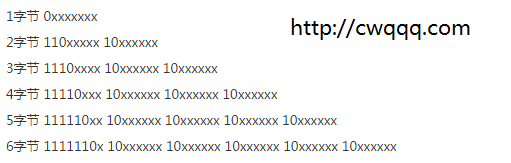# lua匹配UTF-8中文汉字

lua5.3虽然支持utf-8，但是自带的string库不支持汉字的处理，而且lua的正则实现也比较鸡肋，很难匹配中文。所以文章讨论UTF-8字符集，中文汉字的表示方法，然后说明lua如何匹配UTF-8中文汉字。

### 初识UTF-8

UTF-8是Unicode的一种实现，是一种变长字节编码方式。对于某一个字符的UTF-8编码，如果只有一个字节则其最高二进制位为0；如果是多字节，其第一个字节从最高位开始，连续的二进制位值为1的个数决定了其编码的位数，其余各字节均以10开头。UTF-8最多可用到6个字节。### lua匹配UTF-8汉字

```function filter_spec_chars(s)
local ss = {}
local k = 1
while true do
if k > #s then break end
local c = string.byte(s,k)
if not c then break end
if c<192 then
if (c>=48 and c<=57) or (c>= 65 and c<=90) or (c>=97 and c<=122) then
table.insert(ss, string.char(c))
end
k = k + 1
elseif c<224 then
k = k + 2
elseif c<240 then
if c>=228 and c<=233 then
local c1 = string.byte(s,k+1)
local c2 = string.byte(s,k+2)
if c1 and c2 then
local a1,a2,a3,a4 = 128,191,128,191
if c == 228 then a1 = 184
elseif c == 233 then a2,a4 = 190,c1 ~= 190 and 191 or 165
end
if c1>=a1 and c1<=a2 and c2>=a3 and c2<=a4 then
table.insert(ss, string.char(c,c1,c2))
end
end
end
k = k + 3
elseif c<248 then
k = k + 4
elseif c<252 then
k = k + 5
elseif c<254 then
k = k + 6
end
end
return table.concat(ss)
end
```

### 最后语

2016/10/14 修复中文匹配bug，感谢sishui的提示
2017/3/2 修正临时变量k不起作用的问题，提升了效率

http://www.cnblogs.com/chenwenbiao/archive/2011/08/11/2134503.html